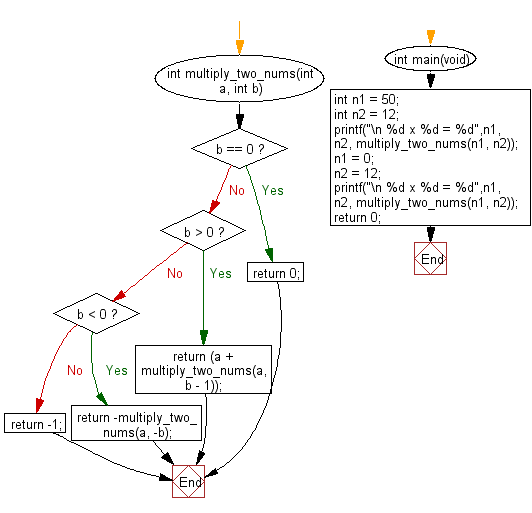﻿ C : Multiply two integers without division, loops

# C Exercises: Multiply two integers without using multiplication, division, bitwise operators, and loops

## C Programming Mathematics: Exercise-20 with Solution

Write a C program to multiply two integers without using multiplication, division, bitwise operators, and loops.

Example 1:
Input: n1 = 50
Input: n2 = 12
Output: 600
Example 2:
Input: n1 = 0
Input: n2 = 12
Output: 0

Sample Solution:

C Code:

``````#include <stdio.h>

int multiply_two_nums(int a, int b) {

/* 0 multiplied with anything gives 0 */
if (b == 0)
return 0;

if (b > 0)
return (a + multiply_two_nums(a, b - 1));

if (b < 0)
return -multiply_two_nums(a, -b);

return -1;
}

int main(void)
{
int n1 = 50;
int n2 = 12;
printf("\n %d x %d = %d",n1, n2, multiply_two_nums(n1, n2));
n1 = 0;
n2 = 12;
printf("\n %d x %d = %d",n1, n2, multiply_two_nums(n1, n2));
return 0;
}
```
```

Sample Output:

```50 x 12 = 600
0 x 12 = 0
```

Flowchart:C Programming Code Editor:

Improve this sample solution and post your code through Disqus.

What is the difficulty level of this exercise?

Test your Programming skills with w3resource's quiz.

﻿